Screenshots

•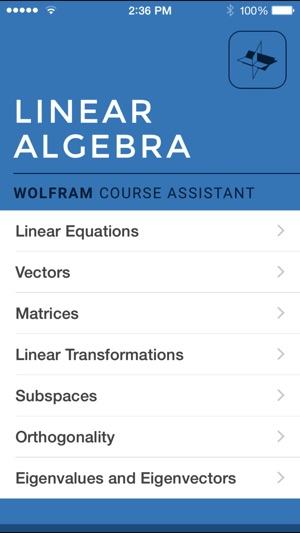•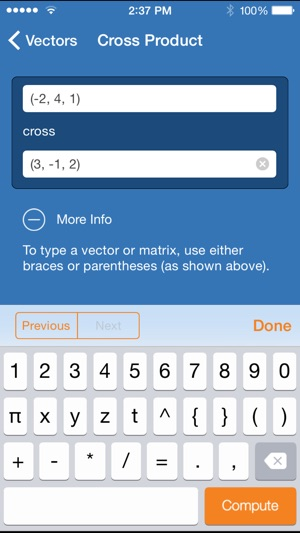•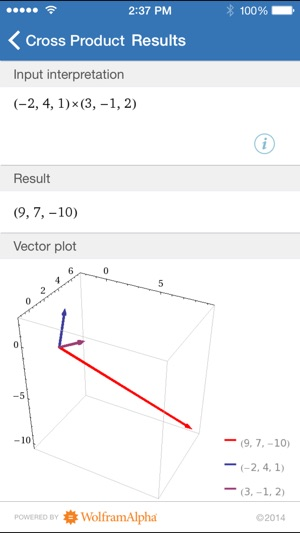•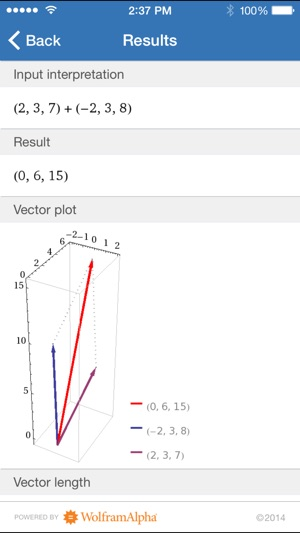•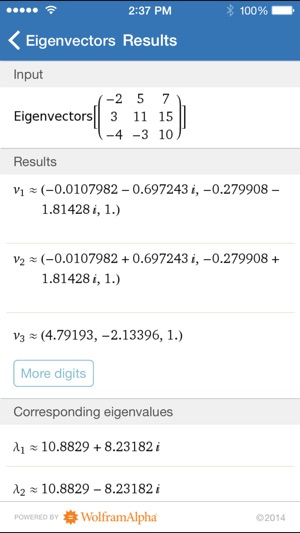Description

Studying linear algebra? Then you need the Wolfram Linear Algebra Course Assistant. This definitive app for linear algebra—from the world leader in math software—will help you work through your homework problems, ace your tests, and learn linear algebra concepts. Forget canned examples! The Wolfram Linear Algebra Course Assistant solves your specific algebra problems on the fly.

This app covers the following topics applicable to linear algebra:

- Solve an equation or a system of equations
- Add or subtract any two vectors
- Find the cross product or dot product for two vectors
- Find the dimensions, transpose, adjugate, rank, inverse, determinant, and reduced row echelon form of a matrix
- Calculate a matrix product
- Add and subtract matrices
- Compute linear transformations
- Determine subspaces, including row space, column space, and null space
- Find the characteristic equation, eigenvalues, and eigenvectors of a matrix

The Wolfram Linear Algebra Course Assistant is powered by the Wolfram|Alpha computational knowledge engine and is created by Wolfram Research, makers of Mathematica—the world's leading software system for mathematical research and education.

The Wolfram Linear Algebra Course Assistant draws on the computational power of Wolfram|Alpha's supercomputers over a 3G, 4G, or Wi-Fi connection.

Version 1.3.1

- Bug fixes

Ratings and Reviews

1.0 out of 5
1 Rating

1 Rating

Floyd2645 ,

Get the main wolframalpha app instead

There's no reason for this app to cost more than the main wolframalpha app while providing less functionality.

NicoleBrewer ,

Because this app was entitled "course assistant" I thought there might be some tutorials or other learning aids. This app is just like any online matrix calculator. The format is very nice, but I downloaded the app in pursuit of a learning tool so it doesn't serve my purpose. The title and description of the app are misleading.

However, if you just want to check your homework with a calculator, I would consider this app highly rated.

Ali Alba ,

Not useful

I have used Wolfram apps before (calculus 1 & 2) and they are helpful unlike this one. To get the answer for one Q you have to do it piece by piece which is stressful. And if u get the right answer there is no STEPS for most of them. Does not worth the money I paid for this app 😖 I would give a one star but I think the spent time working on this app so I will give 2 stars to improve it.

Information

Seller
Wolfram Group LLC
Size
13.3 MB
Category
Education
Compatibility

Requires iOS 9.3 or later. Compatible with iPhone, iPad, and iPod touch.

Languages

English

Age Rating
Rated 4+
© 2017, Wolfram Alpha, LLC.
Price
\$4.99

Supports

•